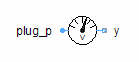Rigid Body - MapleSim Help

Rigid Body

Center of mass (CoM) frame with associated mass and inertia matrixDescription A Rigid Body component is shown schematically in the diagram below. The ground is assumed to be an inertial reference frame and the motion of the body is tracked relative to the ground frame.  When a new rigid body is created, a reference outboard frame (${\mathrm{frame}}_{a}$) is created at the center of mass, C, of the body. The inertia matrix is then specified in terms of its moments and products about C, resolved in components along the local x, y, and z axes at C. If the the option to treat the rigid body as a varying point mass is selected, these products and moments are ignored.To treat the rigid body as a varying point mass, select the Variable point mass parameter. This enables the vMass connection, which allows you to connect a real signal to vMass. The mass of the rigid body will change according to the value of the signal connected to vMass.Connections

 Name Description ${\mathrm{frame}}_{a}$ Rigid body center of mass frame $\mathrm{vMass}$ Variable point mass portParameters

Note:  In the descriptions below, inboard frame refers to the implicit global inertial frame and outboard frame refers to the fixed frame of the Rigid Body.

 Symbol Default Units Description Modelica ID Variable point mass false $-$ If true, the rigid body is treated as a variable point mass (no rotational inertia) useVariableMass m m $\mathrm{kg}$ The mass of the rigid body Mass $\stackrel{}{\left[I\right]}$ $\left[\begin{array}{ccc}{10}^{-6}& 0& 0\\ 0& {10}^{-6}& 0\\ 0& 0& {10}^{-6}\end{array}\right]$ $\mathrm{kg}\cdot {m}^{2}$ The inertia matrix for the rigid body, expressed in the local center of mass frame Inertia ${\mathrm{Type}}_{v}$ $\mathrm{Inboard}$ - Indicates whether the initial velocity is expressed in the inboard or outboard frame. VelType ${\mathrm{Type}}_{\mathrm{θ}}$ $\left[\begin{array}{ccc}1& 2& 3\end{array}\right]$ - Indicates the sequence of body-fixed rotations used to describe the initial orientation of the center of mass frame. For example, $\left[1,2,3\right]$ refers to sequential rotations about the x, then y, and then z axis (123 - Euler angles) RotType ${\mathrm{Type}}_{\mathrm{ω}}$ $\mathrm{Euler}$ - Indicates whether the initial angular velocity is expressed in the inboard or outboard frame.  If Euler is selected, the initial angular velocities are assumed to be the direct derivatives of the Euler angles. AngVelType ${\mathrm{IC}}_{r,v}$ Ignore - Indicates whether MapleSim ignores, tries to enforce, or strictly enforces the translational initial conditions MechTranTree ${\stackrel{&conjugate0;}{r}}_{0}$ $\left[\begin{array}{ccc}0& 0& 0\end{array}\right]$ $m$ Initial displacement of the center of mass frame at the start of the simulation. These values are expressed along the x-, y- and z-axis of the inboard frame respectively InitPos ${\stackrel{&conjugate0;}{v}}_{0}$ $\left[\begin{array}{ccc}0& 0& 0\end{array}\right]$ $\frac{m}{s}$ Initial velocity of the center of mass frame at the start of the simulation. These values are expressed along the x-, y- and z-axis of the inboard frame respectively InitVel ${\mathrm{IC}}_{\mathrm{θ},\mathrm{ω}}$ Ignore - Indicates whether MapleSim ignores, tries to enforce, or strictly enforces the rotational initial conditions MechRotTree ${\stackrel{&conjugate0;}{\theta }}_{0}$ $\left[\begin{array}{ccc}0& 0& 0\end{array}\right]$ $\mathrm{rad}$ Initial rotation of the center of mass frame at the start of the simulation, based on the ${\mathrm{Type}}_{\mathrm{\theta }}$ parameter values InitAng ${\stackrel{&conjugate0;}{\omega }}_{0}$ $\left[\begin{array}{ccc}0& 0& 0\end{array}\right]$ $\frac{\mathrm{rad}}{s}$ Initial velocity of the center of mass frame at the start of the simulation, based on the ${\mathrm{Type}}_{\mathrm{ω}}$ parameter values InitAngVel Use quaternion internally false - Indicates whether the 3-D rotations will be represented as a four parameter quaternion or as three Euler angles.  Regardless of the setting, the initial conditions are specified with Euler angles. useQuatsSee Also